Select Page

# MCQ in English Application of Derivatives Solutions for CBSE Maths 12 Science

MCQ in English Application of Derivatives Solutions for CBSE Maths 12 Science to enable students to get Solutions in a narrative video format for the specific question.

Expert Teacher provides MCQ Application of Derivatives Solutions for CBSE Maths 12 Science through Video Solutions in English language. This video solution will be useful for students to understand how to write an answer in exam in order to score more marks. This teacher uses a narrative style for a question from Application of Derivatives not only to explain the proper method of answering question, but deriving right answer too.

Please find the question below and view the Solution in a narrative video format.

Question:

Solution Video in English:

You can select video Solutions from other languages also. Please check Solutions in ( Hindi )

## Similar Questions from CBSE, 12th Science, Maths, Application of Derivatives

Question 1 : The radius of an air bubble is increasing at the rate of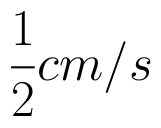. At what rate is the volume of the bubble increasing when its radius is 1 cm? (View Answer Video)

Question 2 : Find the maximum value of in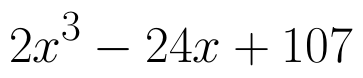the interval [1,3]. find the maximum value of the same function in [-3,-1]. (View Answer Video)

Question 3 : A balloon, which always remains spherical has a variable radius. Find the rate at which its volume is increasing with the radius when the later is 10 cm.  (View Answer Video)

Question 4 : The normal to the curve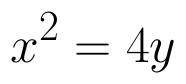passing (1, 2) is: (View Answer Video)

Question 5 : The line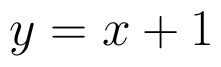is a tangent to the curve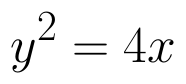at the point. (View Answer Video)

### Determinant

Question 1 : If A is a symmetric matrix, then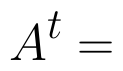? (View Answer Video)

Question 2 : If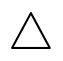=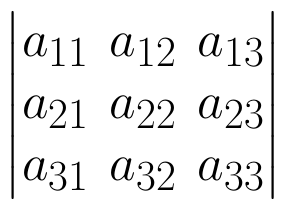and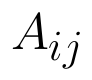is Cofactor of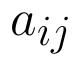, then the value ofis given by (View Answer Video)

Question 3 : If A =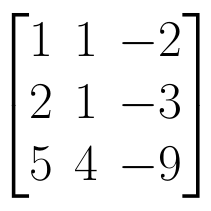, find | A |. (View Answer Video)

Question 4 :  Find the area of the triangle with vertices at the points (2, 7), (1, 1), (10, 8). (View Answer Video)

Question 5 : Using the properties of determinants, evaluate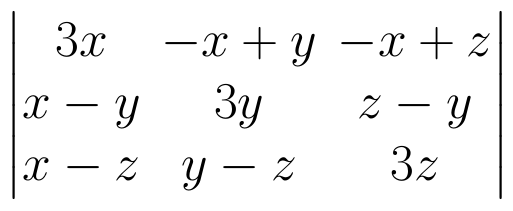. (View Answer Video)

### Continuity and Differentiability

Question 1 : Findfor the function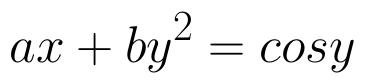. (View Answer Video)

Question 2 : If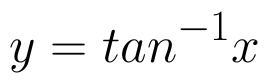, find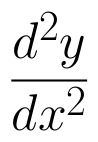in terms of y alone. (View Answer Video)

Question 3 : Findfor the function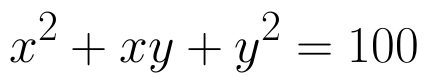. (View Answer Video)

Question 4 : Differentiate the function w.r.t.x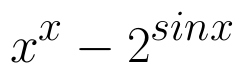. (View Answer Video)

Question 5 : Differentiate the function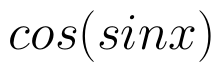with respect to x. (View Answer Video)

### Matrices

Question 1 : Let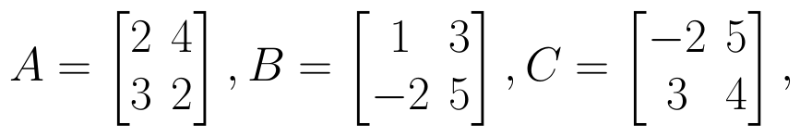Find A + B. (View Answer Video)

Question 2 : Compute: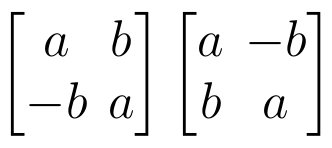. (View Answer Video)

Question 3 : Find the value of X, if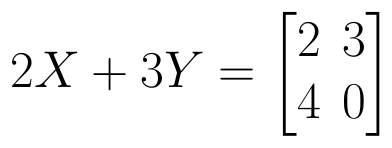and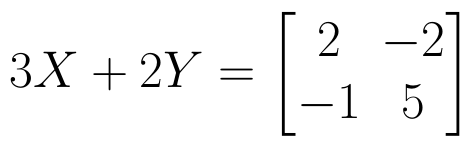. (View Answer Video)

Question 4 : Given,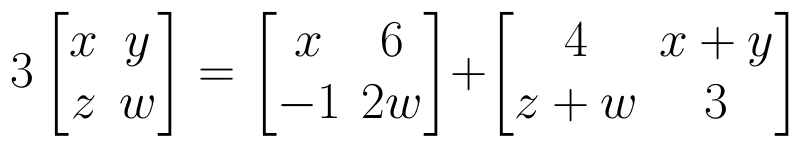, find the value of w. (View Answer Video)

Question 5 : Find the value of y,  from the equation: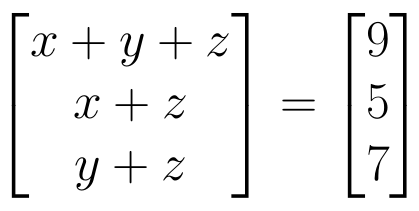. (View Answer Video)